# Activity: Lengths of Leaves

In this Activity we will investigate the lengths of leaves and work out the mean (average) length.

You are also going to learn a method to find an estimate of the mean length.

The first thing you need to do is to find some leaves ...

## What kind of leaves?

You can use leaves from any tree or plant; but if it's a tree, then choose one that is easy to reach (we don't want any accidents), and don't take your neighbor's leaves without asking! Also it's best to use simple leaves. Don't try to use leaves with strange shapes. The kind of leaves you see in the photograph below are ideal.

#### Rules

Some of the rules you might want to consider are:

• I must choose all my leaves from the same tree or plant.
• I must make sure the leaves are whole - broken leaves just won't do.
• I must try to get a random sample of leaves - not all big ones and not all small ones.

The following photograph shows four leaves of different sizes that I collected from a tree in my garden: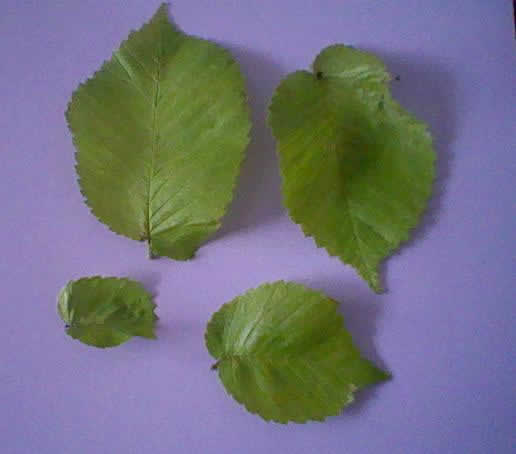You will see that some leaves are small and some are larger.
The next thing you need to decide is how many leaves to pick and how to get a random sample.

## How many?

You could pick as many as you like. Ideally you would use all the leaves on the tree, but that would be rather a large number to measure, and your parents might not be very happy if you stripped the tree bare!

So let's try 100 leaves.

## How to get a random sample?

Here is one way you could get a random sample:

• Try taking all the leaves on one branch of the tree. After you've discarded any that are broken, then see how many there are. If there are less than 100, then get the leaves from another branch until you have more than 100. Then write a different number (starting with 1) on each leaf with a marking pen.
• Write the same numbers on small pieces of paper.
• Fold the pieces of paper.
• Put them into a hat and mix them up.
• Draw 100 pieces of paper (without looking) from the hat. The first 100 pieces of paper you draw would give you the numbers of the leaves to use in the experiment.
The next thing you need to decide is how to measure the leaves, and
how accurate your measurements should be:

## How to measure?

Keeping your leaves flat, use a ruler to measure the length of each leaf from the pointy part at one end of the leaf to the point where the leaf joins the stalk at the other end. Maybe your leaves bend a bit, but don't follow the main rib of the leaf as this would make measurement too difficult.

Just measure in a straight line as shown in the following diagram: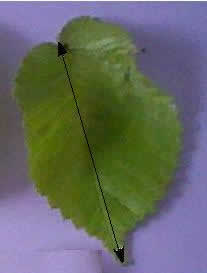## How accurate?

### You should measure the length of each leaf to the nearest millimeter.

Measure the length of each leaf to the nearest millimeter and record you results in a table, as follows:

(5 columns of 20 each equals 100 measurements)

 Length (mm) Length (mm) Length (mm) Length (mm) Length (mm) 1 2 3 4 5 6 7 8 9 10 11 12 13 14 15 16 17 18 19 20 Sums:

Add up each column, then add those sums together for the grand total:

_______ + _______ + _______ + _______ + _______ = _________

 Grand Total:

## Finding the Mean

Now you should be able to calculate the mean length of your leaves. Simply divide the sum of lengths by 100 .

 Mean (= Grand Total / 100):

## Is There Another Way?

There is a way to estimate the mean length by grouping your results.

This makes the calculation quicker, but is not as accurate.

### Example

I measured the lengths of 100 leaves each to the nearest millimeter and grouped the results into groups of size 10 millimeters using a tally and constructed a frequency table, as follows:

 Length (mm) Tally Number of leaves (frequency) 20 - 29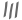3 30 - 39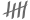8 40 - 4915 50 - 59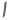26 60 - 6923 70 - 7916 80 - 89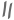7 90 - 992 Total 100

If this is not clear, then let me explain:

The three shortest leaves in my sample have lengths 22 mm, 25 mm and 27 mm. These are all between 20 mm and 29 mm, so each of them adds a tally mark for the group 20 - 29.

So there are three tally marks altogether () in the group 20 - 29, and the frequency for this group is 3 (i.e. there are 3 leaves in this group).

Similarly, there are eight leaves whose lengths are between 30 mm and 39 mm, so there are 8 tally marks for this group and the frequency is 8.

And so on.

## Once you've grouped your lengths, how can you estimate the mean?

What we do is we assume all leaves in a group have the same length, which is the average for that group. This value is called the midpoint of the group and is simply found by taking the average (mean) of the smallest and greatest lengths in that group.

For example:

The midpoint for the group 20 - 29 is (20 +29)/2 = 49/2 = 24.5

The midpoint for the group 30 - 39 is (30 +39)/2 = 69/2 = 34.5

etc

So, returning to my example, I am going to assume that there are 3 leaves with a mean length of 24.5 mm, 8 leaves with a mean length of 34.5 mm, 15 leaves with a mean length of 44.5 mm , etc. I can then work out my estimate of the mean length from a frequency table, as follows:

 Length (mm) Midpoint x Frequency f f × x 20 - 29 24.5 3 73.5 30 - 39 34.5 8 276.0 40 - 49 44.5 15 667.5 50 - 59 54.5 26 1,417.0 60 - 69 64.5 23 1,483.5 70 - 79 74.5 16 1,192.0 80 - 89 84.5 7 591.5 90 - 99 94.5 2 189.0 Σf = 100 Σ(f × x) = 5,890

So the estimate of the mean length = Σ(f × x)/Σf = 5,890/100 = 58.9 mm

This value differed a little from the exact value of the mean that I obtained earlier. It's important to understand that this is just an estimate, but it can be much quicker to calculate.

### Try using the method for your sample and see how good an estimate you get.

You can use this table. First decide which length groups to use. You may need fewer groups or more groups than we did. For example, if you have a leaf of length 105 mm, then you will need an extra group 100 - 109. Or you might not have any leaves as short as mine, so you may not need the group 20 - 29. Choose the right groups for your results.

 Length (mm) Midpoint x Tally Number of leaves f f × x Total Σf = 100 Σ(f × x) =

Divide Σ(f × x) by Σf to get the mean length:

Σ(f × x) / Σ(f) = ________ / 100 = _________

## Why use an Estimate?

Faster! Easier! (But less accurate.)

It might not have made much difference in our example, with only 100 leaves, but it could be a useful method to use when the number in a sample is much much larger.